# Two cars

Two cars started against each other at the same time to journey long 293 km. First car went 41 km/h and second 41 km/h.

What distance will be between this cars 20 minutes before meet?

Result

s =  27.3 km

#### Solution:

$v = 41 + 41 = 82 \ km/h \ \\ s = 293 - (293 + \dfrac{ 20}{60} \cdot 82) = \dfrac{ 20}{60} \cdot 82 = 27.3 \ \text { km }$

Leave us a comment of this math problem and its solution (i.e. if it is still somewhat unclear...):Be the first to comment!#### Following knowledge from mathematics are needed to solve this word math problem:

Do you have a linear equation or system of equations and looking for its solution? Or do you have quadratic equation? Do you want to convert length units?

## Next similar math problems:

1. Three linesAt 6 am, three bus lines are departing from the station. The first line has an interval of 24 minutes. The second line has an interval of 15 minutes. The third line runs at regular intervals of more than 1 minute. The third line runs at the same time as th
2. Kilowatt-hours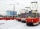If the Lewis family used 648 kilowatt-hours of electricity in 12 days, how many kilowatts hours should they use in 24 days at the same rate of usage
3. Break timeCalculate how many percent of school time is spent on breaks. Calculate data for the whole week.
4. 45th birthdayThis year Mrs. Clever celebrated her 45th birthday. Her three children are now 7.11 and 15 years old. In how many years will Mrs. Clever's age be equal to the sum of her children's years?
5. Coal mine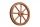The towing wheel has a diameter of 1.7 meters. How many meters does the elevator cage lower when the wheel turns 32 times?Find the radius of the circle with area S = 200 cm².
7. Floating of wood - Archimedes lawWhat will be the volume of the floating part of a wooden (balsa) block with a density of 200 kg/m3 and a volume of 0.02 m3 that floats in alcohol? (alcohol density is 789 kg/m3)
8. Cheops pyramid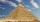The Pyramid of Cheops is a pyramid with a square base with a side of 233 m and a height of 146.6 m. It made from limestone with a density of 2.7 g/cm3. Calculate the amount of stone in tons. How many trains with 30 twenty tons wagons carry the stone?
9. Common divisorsFind all common divisors of numbers 30 and 45.
10. Numbers divisionWith what number should be divided mixed number 2 3/4 to get 11/12?
11. Cut trees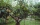There are 1,200 trees in the grove, of which 55 percent are deciduous, the remaining coniferous. Workers cut down 35 percent a) trees, b) deciduous trees, c) coniferous trees. How many trees now have this grove?
12. Barrel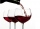Barrel of wine is filled by 9/14. What part of the barrel will remain filled when 1/4 of the wine we pour from the barrel?
13. Year 2020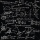The four-digit number divided by 2020 gives a result of 1, **. (Can not be in form 1,*0. ) Write all the options.
14. The cuboidThe cuboid is filled to the brim with water. The external dimensions are 95 cm, 120 cm, and 60 cm. The thickness of all walls and the bottom is 5 cm. How many liters of water fit into the cuboid?
15. Math testIn mathematics, there were 25 problems of three kinds: light 2 points, medium 3 points, heavy 5 points, the best score is 84 points. How many points did Jane have when she solved all the easy examples, half medium and one-third difficult?
16. In centerWhat number lies in the center between a quarter of a fifth and a half of a third on the number axis?
17. Dividing moneyVilem, Cenek, and Edita divided the money they earned by spreading the leaflet. Vilem got 240 CZK more than Cenek and twice more than Edita. Edita got 400kc less than Vilem.